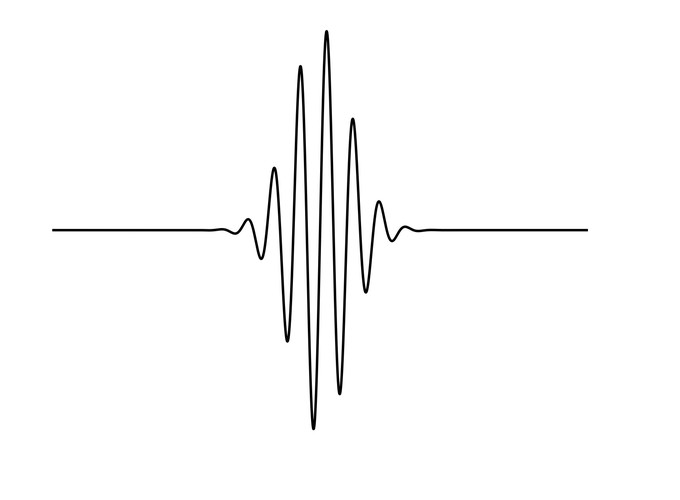# Derivation of the Transform Limit

Let us define the dispersion of a function about zero as,

$$D_0(f)=\int_{-\infty}^\infty x^2 |f(x)|^2 dx$$

Where $f(x)$ is some normalized square integrable function. In this case we’ll use a gaussian which is defined as,

$$f(x)\propto e^{-x^2/2\sigma^2}$$

This could be, for instance, the amplitude of the electric field of some laser pulse. We also know that the fourier transform of a gaussian is still a gaussian, i.e.

$$\mathcal{F}[f(x)](k)=\hat{f}(k)\propto e^{-2\pi^2\sigma^2k^2}$$

We can relate the two dispersions to one another by multiplying them together, i.e.,

$$D_0(f)D_0(\hat{f})=\sigma^2_x\sigma^2_k$$

Remember that we know

$$\sigma^2=\int_{-\infty}^{\infty}x^2\frac{e^{-x^2/2\sigma^2}}{\sqrt{2\pi\sigma^2}}dx$$

Using this fact we can say that

$$\sigma^2_x=\frac{\sigma^2}{2}$$

And

$$\sigma^2_k=\frac{1}{8\pi^2\sigma^2}$$

Which means that our dispersion relation is

$$D_0(f)D_0(\hat{f})=\frac{1}{16\pi^2}$$

We can relate this to the FWHM of our pulse in either the $x$ or $k$ domain using the fact that $\text{FWHM}=2 \sqrt{2\ln 2} \sigma$,

$$\Delta x \Delta k=\frac{2\ln2}{\pi}\approx0.441$$

Where $\Delta x$ is the FWHM of the square of $f(x)$ in the $x$ domain. For instance, if $f(x)$ was the electric field of our pulse than $|f(x)|^2$ would be the intensity that we would measure of the pulse and $\Delta x$ would be the measured FWHM of the intensity of the pulse.

We can make this more concrete by saying that we measured the frequency of the pulse in cm$^{-1}$ and the duration in fs,

$$\Delta t (\text{fs}) \Delta \nu (\text{cm}^{-1})\left(\frac{ 2.99 \times 10^{-5}\text{ cm}}{\text{fs}}\right)=0.441$$

We can rearrange this to be

$$\Delta t (\text{fs}) \Delta \nu (\text{cm}^{-1})=1470\text{ fs cm}^{-1}$$

Or

$$\Delta t (\text{ps}) \Delta \nu (\text{cm}^{-1})=15\text{ ps cm}^{-1}$$

##### David P. Hoffman
###### Staff Optical Engineer @ 10x Genomics

I’m a scientist/engineer who builds robust, high-performance optical systems and loves data and python. Views are my own (whose else’s would they be?)